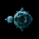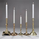English
TradingView
FX:EURUSD   Euro / U.S. Dollar
160 views
160
Update to the previous version of Arbitrary Price Pivot tool. Here we have specific pivot levels based on the Standard Distribution Deviations. You will see three levels of each deviation greater than 97.7% (2.0). For example, standard deviation 3.09 has a ratio of 99.99% certainty price will return to the mean. So we have 3.09, 6.18 and 9.27...three levels of that particular deviation. If price breaks through 3.09 it most likely will continue through to the next level, 6.18 and perhaps 9.27. All relative to time frame used.
Comment: Aribitrary Price Pivot script posted.//Arbitrary Price Pivots/StdDev 3; ^, standard distribution deviations, set your own pivot levels
study("Arbitrary Price Pivots/StdDev3^; ",overlay=true)

p = input(000.0000,title="Pivot")
p0 = input(1.0050, title="p0")
p1 = input(1.0100, title="p1")
p2 = input(1.0128, title="p2")
p3 = input(1.0165, title="p3")
p4 = input(1.0200, title="p4")
p5 = input(1.0233, title="p5")
p6 = input(1.0258, title="p6")
p7 = input(1.0309, title="p7")
p8 = input(1.0400, title="p8")
p9 = input(1.0466, title="p9")
p10 = input(1.0516, title="p10")
p11 = input(1.0600, title="p11")
p12 = input(1.0618, title="p12")
p13 = input(1.0699, title="p13")
p14 = input(1.0774, title="p14")
p15 = input(1.0927, title="p15")
plot(p,"Pivot",color=green)
plot(p*p0, title="38.2%, 0.50stddev", color=aqua)
plot(p/p0, title="38.2%, 0.50stddev", color=aqua)
plot(p*p1,"84%, 1.00stddev",color=white)
plot(p/p1,"84%, 1.00stddev",color=white)
plot(p*p2,"90%, 1.28 stddev",color=lime)
plot(p/p2,"90%, 1.28 stddev",color=lime)
plot(p*p3,"95%, 1.65 stddev",color=olive)
plot(p/p3,"95%, 1.65 stddev",color=olive)
plot(p*p4,"97.7%, 2.00 stddev",color=blue)
plot(p/p4,"97.7%, 2.00 stddev",color=blue)
plot(p*p5,"99%, 2.33 stddev",color=yellow)
plot(p/p5,"99%, 2.33 stddev",color=yellow)
plot(p*p6,"99.5% 2.58 stddev",color=orange)
plot(p/p6,"99.5% 2.58 stddev",color=orange)
plot(p*p7,"99.99% 3.09 stddev",color=red)
plot(p/p7,"99.99% 3.09 stddev",color=red)
plot(p*p8,"4.0, 2.00 stddev 2^",color=blue)
plot(p/p8,"4.0, 2.00 stddev 2^",color=blue)
plot(p*p9,"4.66, 2.33 stddev 2^",color=yellow)
plot(p/p9,"4.66, 2.33 stddev 2^",color=yellow)
plot(p*p10,"5.16, 2.58 stddev 2^",color=orange)
plot(p/p10,"5.16, 2.58 stddev 2^",color=orange)
plot(p*p11,"6.18, 3.09 stddev 2^",color=red)
plot(p/p11,"6.18, 3.09 stddev 2^",color=red)
plot(p*p12,"6.0, 2.00 stddev 3^",color=blue)
plot(p/p12,"6.0, 2.00 stddev 3^",color=blue)
plot(p*p13,"6.99, 2.33 stddev 3^",color=yellow)
plot(p/p13,"6.99, 2.33 stddev 3^",color=yellow)
plot(p*p14,"7.74, 2.58 stddev 3^",color=orange)
plot(p/p14,"7.74, 2.58 stddev 3^",color=orange)
plot(p*p15,"9.27, 3.09 stddev 3^",color=red)
plot(p/p15,"9.27, 3.09 stddev 3^",color=red)
Replycoondawg71
Thank you for the script .. .but when I add it to the chart through pine editor it doesn't show anything.
ReplyEde
It was my fault. Thank you very much coondawg71 .
ReplyEde
Sorry pal, I never saw the comment. I will post the script shortly.
Reply
EN English
EN English (UK)
EN English (IN)
DE Deutsch
FR Français
ES Español
IT Italiano
PL Polski
SV Svenska
TR Türkçe
RU Русский
PT Português
ID Bahasa Indonesia
MS Bahasa Melayu
TH ภาษาไทย
VI Tiếng Việt
JA 日本語
KO 한국어
ZH 简体中文
ZH 繁體中文
AR العربية
HE עברית